# Hindi Poem Generation

Indian poetry has a rich and long history dating back a millennium. People from varying demographics, with different spoken languages and traditions, have commonly used poetry as a medium to express emotion. Shayaris, originally written in Urdu, are short poems consisting of a few couplets. Today, shayaris are popularly used to express different emotions ranging from love and devotion to sadness and frustration. Shayaris can be fun and parodic or can inspire and motivate. Acknowledging the importance of shayaris in Indian culture, ShareChat has provided its users with a platform to share original shayaris that can entertain and inspire the readers. Typical examples of shayaris popular on ShareChat are given below.

Writing shayaris like these is inherently a creative process. Creativity, like intelligence, is intricately linked to the human experience. Today, AI solutions are used to solve a variety of problems, but creativity may be the ultimate frontier for artificial intelligence. In this article, we will look at how state-of-the-art Natural Language Generation techniques can be used to excel in the creative exercise of shayari generation.

# Natural Language Generation

Natural Language Generation (NLG) is the sub-field of artificial intelligence and computational linguistics that is concerned with the construction of computer systems that can produce understandable texts in English or other human languages from some underlying non-linguistic representation of information.

— Page 1, Building Applied Natural Language Generation Systems, (1997)

Automatic Natural Language Generation (NLG) has had a long tradition in the Computational Linguistics community, but the recent advances in deep learning have given it a new impetus. Its importance in the modern world cannot be overstated. If we want our AI systems to understand and interact with the world, they have to be fluent in the foremost form of human communication — natural language.

NLG tasks can be broadly classified into two types:

1. Unconditional text generation, where we generate fluent sentences that follow the distribution of sentences in natural language.
2. Conditional text generation, where we generate a sentence which expresses desired content or stylistic properties, typically from a given content and/or style representation.

# Shayari Generation as a NLG Task

We investigated state-of-the-art methods in NLG for the task of unconditional Hindi Shayari generation. Popular methods for unconditional text generation are typically based on:

1. n-gram counts
2. Feed-forward neural networks
3. Recurrent Neural Networks
4. Transformers
5. Generative Adversarial Networks

For our task, we started with simple LSTMs and then went onto evaluate deep transformer-based architectures and GANs. We observed the benefits that come from pre-training and studied how using GANs can help improve the quality of generated samples. We will describe each of these approaches in detail in the following sections. The trained models can be downloaded from here. We also provide a script that can be used to generate shayaris here.

Below, we look at how recurrent and transformer-based architectures trained using maximum likelihood estimation (refer appendix for more details on MLE-trained models) perform in the task of shayari generation.

## Recurrent Language Models

Recurrent language models are a natural choice to create a baseline for any NLG task. If you are not familiar with how RNNs can be used for language modeling, please refer to this awesome explanation by Andrej Karpathy. For our experiments, we used 1–2 layer LSTMs with < 10M parameters trained in a word-level many-to-many fashion. We use perplexity to evaluate the performance of the trained models. When trained on a dataset of 120K shayaris, our model achieved a test perplexity of 174. We then experimented with pre-training on the 400 MB Hindi Wikipedia corpus (cleaned using wikiextractor) before fine-tuning on the shayari dataset. This helped to reduce the final perplexity on the shayari test set to 104. However, we observed that the model suffered from catastrophic forgetting. The perplexity on the hiwiki test set increased from 270 (before fine-tuning on shayari dataset) to 3640 (after fine-tuning). This behavior is not desirable. Once the model acquires some general syntactic and semantic knowledge about the Hindi language in the pre-training stage, we want it to retain that knowledge during the fine-tuning stage.

## GPT-2

Recently, transformers have replaced LSTMs for the top spot in language modeling performance. For our experiments, we used OpenAI’s GPT-2-small model that consists of 12 transformer decoder blocks, each with 12 attention heads. There are great resources if you want to learn more about GPT-2, so I’m not going to go into the details here. For shayari generation, we trained GPT-2 on a corpus constructed from Hindi Wikipedia and Hindi commoncrawl dumps. The Hindi language dumps of the form hi.<timestamp>.raw.xz were extracted, combined, and deduplicated. The corpus thus formed was 19 GB in size, big enough to pre-train the 124M parameter GPT-2-small model. The model was then fine-tuned on the shayari data.

Throughout the fine-tuning process, we observed how the performance of the model degraded on the cc+hiwiki dataset, that was used for pre-training. We observed that the problem of catastrophic forgetting for the GPT-2 model isn’t as severe as it was for the LSTM case (Fig. 1).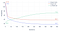Fig. 1: Perplexity metrics during fine-tuning iterations (lower is better)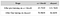GPT-2 Experiments. Metric used is test set perplexity (lower is better).

# Decoding

Once the model is trained, we need to decide the method used to “decode” the output probability distributions to generate samples. Commonly used methods are:

Argmax sampling. Given the probability distribution over vocabulary at each timestep, we generate the token which is assigned the maximum probability by the model. This process is deterministic i.e. if the model parameters are fixed, we get the same generation for multiple tries with the same prompt.

Low-Temperature Sampling. To enable the model to generate novel samples on every trial, we can perform randomly sample the token from the categorical distribution at the model’s output. However, this doesn’t work well in practice and the samples are typically noisy and of lower quality. To overcome this we can use a temperature parameter to control the entropy of the softmax’s output. A temperature lower than 1 will make the categorical distribution at the model’s output more confident and have less entropy. With the addition of the temperature parameter T, the softmax can be re-estimated as:

where t_i is the ith token and z_j is the logit corresponding to the jth token in a vocabulary of size V. If we set T = 1, we can recover the original softmax and as T approaches 0, we recover argmax sampling.

Beam Search. If our goal is to generate samples for which the model assigns the maximum probability, the generation process suffers from time complexity that grows exponentially with sequence length. A more efficient way to do this, albeit sub-optimally, is beam search. The beam search algorithm reduces the search space for possible candidates using a fixed beam width, and reduces the time complexity from exponential to linear in sequence length.

Although these methods are intuitive and popular, they are not perfect. Argmax samples lack the ability to recover from the mistakes that the model makes at previous timesteps. With low-temperature sampling, the higher sample quality comes at a cost of decreased diversity. Beam search often to degenerate and repetitive samples. Top-k sampling was proposed to alleviate some of these problems by only sampling from the top k most probable tokens at each timestep. However, choosing a single k for all sampling steps becomes problematic.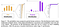Problem with choosing a single k. Source: https://arxiv.org/pdf/1904.09751.pdf

Hence for our purpose, we use Nucleus Sampling, where sampling is performed from the top-p portion of the probability mass, effectively expanding and contracting k dynamically. Below, we show some samples obtained using nucleus sampling from our LSTM and transformer models:

Shayari samples from LSTM model trained directly on shayari data:

`इश्क़ करने का शौक नहीं है! बस इतना समझ लो.देखिये किस कदर रोया है, कोई कहता है प्यार सज़ा बन जाता है।`

Shayari samples from LSTM model trained on shayari data after pretraining on hiwiki:

`इश्क़ करने का हक है तुम्हे, पर नाराजगी में कहीं ये मत भूल जाना कीदेखिये किस कदर करोगे ? हमने भी मुस्कुरा कर कहा. मोहब्बत ऐसी हो गयी.`

For GPT-2, along with nucleus sampling, we also experimented with different temperatures during the sampling process. We observed that reducing temperature did give us better quality samples, but at the cost of reduced diversity. Below, we report test metrics that evaluate both quality (BLEU, NLL_{oracle}) and diversity (Perplexity). As we reduce temperature, quality improves but diversity worsens. We also observed that there is a limit to which we can observe improvements in quality by lowering the temperature. The quality improvement that we achieve upon reducing the temperature to 0.5 is not clear, and is not worth the reduced diversity of generated shayaris.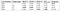GPT-2 experiments with different temperatures.

To generate your own samples, clone this repository and follow the environment setup guide.

Shayari samples from GPT-2 model with temperature set to 1:

`\$ python main.py --model_path models/mle-model/ --tokenizer_path tokenizer/ --top_p 0.9 --temperature 1>>> इश्क़ करने का100%|████████████████████████████| 100/100 [00:01<00:00, 53.53it/s]इश्क़ करने का मौका ही ना मिलेमुझ को तो अब तेरी जिद्द भी ठुकराने का मौका ही ना मिले>>> देखिये किस कदर100%|████████████████████████████| 100/100 [00:01<00:00, 62.15it/s]देखिये किस कदर प्यार है,ना जाने किस किस की तलाश है,जाने किस किस का क़र्ज़ है,जिसका क़र्ज़ है उसी का इंतज़ार है`

Shayari samples from GPT-2 model with temperature set to 0.7:

`\$ python main.py --model_path models/mle-model/ --tokenizer_path tokenizer/ --top_p 0.9 --temperature 0.7>>> इश्क़ करने का100%|████████████████████████████| 100/100 [00:01<00:00, 62.98it/s]इश्क़ करने का मन है,मुझे तुमसे प्यार करना है,तुम मत पूछो कितना दर्द होता है,  बस एक बार तुमसे मिलने कीकोशिश करना है.>>> देखिये किस कदर100%|████████████████████████████| 100/100 [00:01<00:00, 63.24it/s]देखिये किस कदरइश्क में डूबे हो तुमदिल में उतर केदेखना चाहते हो तुम`

Although we achieve fairly good results with nucleus sampling, the MLE-based training procedure itself has some fundamental limitations which cannot be fixed by just a clever decoding strategy.

# Problems with MLE training

Although the MLE training approach is widely used, the resultant language models have some drawbacks:

1. Exposure Bias: Because of teacher forcing (see appendix), the training process turns out to be very brittle because the model is only exposed to the actual data distribution during training. During testing, the model is expected to predict the next word by using words previously drawn from the model distribution as inputs. This discrepancy makes it difficult for the model to recover from mistakes it makes during testing time, and the errors quickly accumulate.
2. Word level loss: LMs are trained using word-level loss functions (cross-entropy), but the evaluation is done using human evaluation or sequence-level metrics such as BLEU which measure the n-gram overlap between the generated samples and the reference text. These sequence-level metrics are not differentiable to facilitate training using gradient descent.
3. Overgeneralization: When we minimize cross-entropy (or D_KL(p_{data} ‖ p_G) equivalently, see appendix) with a model that is under parametrized, the model distribution (p_G) thus obtained overestimates the entropy of the true data distribution. This results in the model assigning a higher probability to samples that are implausible and of low quality (mean-seeking behavior).

The train-test time discrepancy thus introduced can result in unexpected model behavior during test time. RL or GAN-based approaches aim to avoid both of these problems by only using samples from the policy/generator model during training (no exposure bias) and policy-gradient based/adversarial training (no word-level loss and overgeneralization). In the following sections, we discuss how these approaches differ from MLE-trained models and how the added complexity affects the training and the quality of generated samples.

# GANs

Generative Adversarial Networks are models that make clever architectural choices that make them suitable for generation tasks. They have two neural network components:

• A generator, that learns to generate plausible samples that resemble samples from the training set.
• A discriminator, that learns to distinguish the generator’s fake data from real data.

The generator’s output is connected directly to the discriminator input. Through backpropagation, the discriminator’s classification provides a signal that the generator uses to update its weights. So effectively, the generator is tasked to generate samples that make it harder for the discriminator to tell the difference.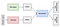Generative Adversarial Network. Source: https://developers.google.com/machine-learning/gan/gan_structure

When training begins, the generator produces obviously fake data, and the discriminator quickly learns to tell that it’s fake. But as training progresses, the generator learns to generate better and better samples that eventually, if all goes well, can fool the discriminator.

This adversarial training setup, where the generator and discriminator simultaneously minimize and maximize the same objective turns out to be equivalent to minimizing the Jensen-Shannon Divergence between the data distribution and generator’s distribution. The GAN objective is given as follows:Eq. 1: GAN objective

Where G and D refer to the generator and discriminator networks respectively. If the discriminator (D(x)) is assumed to be Bayes optimal, we can recover the original JSD objective (see appendix, or for more details, see the original paper). Described in two steps, the goal of GAN training is to

• given a fixed G, estimate parameters for the Discriminator D such that V(G, D) is maximized (called the maximization step), and
• given a fixed D, estimate parameters for the Generator G such that V(G, D) is minimized (called the minimization step).

During each training iteration, the parameters for the networks G and D are estimated alternatively using gradient descent while keeping the other fixed.

Models trained on cross-entropy (or forward KL divergence) are prone to overgeneralization (see appendix). This typically results in generated samples of greater diversity but lesser quality. The GAN objective, on the other hand, minimizes JSD, which can be shown to fall halfway between forward and reverse KL divergence, and thus discourages overgeneralization. As a consequence, samples from GANs are typically of higher quality at the cost of lower diversity. Due to this quality-diversity trade-off, GANs are known to suffer from a problem known as mode collapse where the generator’s distribution tends to collapse to a single mode of the true data distribution, ignoring other modes, resulting in a lack of diversity in the generated samples.

## GANs for text generation

Unlike image GANs, adversarial training can’t be performed trivially for text generation. The discrete text outputs from the generative model make it difficult to pass the gradient update from the discriminative model to the generative model. Text sequences need to be sampled from the generator model distribution before feeding the to the discriminator, and the sampling step is not differentiable. This inhibits the gradients from the discriminator to reach the generator. To solve this problem two solutions have been proposed:

1. REINFORCE: Policy gradients can be used for the generator gradient update. But the use of RL comes with its own drawbacks such as unstable training and large action space, and slow convergence.
2. RL-free approaches. Gumbel-Softmax GAN uses argmax samples from the reparametrized Gumbel-Softmax distribution that allows gradient flow. Other approaches such as Cooperative Training and FM-GAN are also proposed.

Despite the enormous success of transformers in language modeling, recent work on Text GANs often use LSTM networks as generators instead of transformer networks. The reason behind this is that transformers, being completely self-attention based and highly parallelized, are very slow at autoregressive sampling as compared to inference. The inference and sampling times for LSTMs on the other hand, are very similar. The slow sampling makes training the GAN setup with transformer generators impractical. N3DGAN offers a clever solution to this problem.

N3DGAN (No Neural Network as the Discriminator GAN) uses a generator network that can explicitly model p_G, unlike implicit density models that are used as generators especially for vision tasks. Instead of explicitly using a neural network to model the discriminator (D(x)), the D(x) term in the JSD-based GAN objective (Eq. 1) is replaced with a closed-form solution for the optimal discriminator (Eq. 2) that is only dependent on the empirical p̃_{data} and p_G.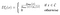Eq. 2: Closed-form solution for the optimal discriminator

Where C refers to the training set. Recall from Eq. 1 that, during the maximization step of a training iteration, we aim to estimate parameters for D such that the objective V(G, D) is maximized, given a fixed G. The closed-form solution given above gives the optimal solution for D that does exactly this, without using a neural network to model the discriminator. Hence, during each training iteration, we obtain D not using gradient descent on an actual neural network, but using this closed-form solution. The optimization objective (Eq. 3) thus obtained (see the paper for more details) only requires examples from the training set (empirical p̃_{data}) and does not require sampling from the generator.Eq. 3: N3DGAN Objective

As autoregressive sampling (sampling from p_G) is not required to optimize the N3DGAN objective, this makes the use of transformer-based generators feasible. Here, it is important to note that the empirical data distribution, denoted by p̃_{data} in the N3DGAN objective, is constant, and non-zero only when x ∈ C (see appendix Eq. 7). This constancy of p̃_{data}(x) for x ∈ C does simplify the objective, but to intuitively understand how the objective differs from standard cross-entropy, we will visualize how the gradients of the losses are different.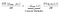Gradient of the cross-entropy loss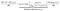Gradient of the N3DGAN loss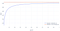Plotting how the multiplier varies with p_G(x). p_{data}(x) = 1/16.

For Cross-Entropy loss, we see the gradient multiplier is constant and independent of p_G(x). However, for the N3DGAN loss, we observe that when p_G(x) is low, the magnitude of the multiplier is small, resulting in a gradient of smaller magnitude. Hence, when the model assigns a low probability to a training sample x, the loss essentially encourages it to ignore the sample. In the case where the model assigns a high probability to a sample, the loss encourages it to assign an even higher probability. This results in the mode-seeking behavior that we also saw while discussing reverse KL divergence (see appendix). As the N3DGAN loss is equivalent to JSD, the properties of reverse KL divergence can be seen here.

## GAN Experiments for Shayari generation

For our experiments, we replaced the cross-entropy loss with the N3DGAN loss in Eq. 3, and fine-tuned the MLE-trained GPT-2 on the shayari data. The results we obtained were as expected — the samples were of significantly better quality, but with loss in diversity. We also experimented with optimizing JSπ (see appendix Eq. 5) with different values of π. This enables us to control the tradeoff between quality (achieved by optimizing reverse KL) and diversity (achieved by optimizing forward KL). We report the results below: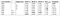N3DGAN experiments with different values of π

With larger values π, the JSD objective helps us to further improve quality without any loss in diversity.

Some shayari samples from the GAN model:

`\$ python main.py --model_path models/n3dgan-model/ --tokenizer_path tokenizer/ --top_p 0.9>>> इश्क़ करने का100%|████████████████████████████| 100/100 [00:01<00:00, 52.25it/s]इश्क़ करने का हुनर हमे सिखाया है, जिस दिल को हमने अपनी रूह में बसाया है.>>> इश्क़ करने का100%|████████████████████████████| 100/100 [00:01<00:00, 61.33it/s]इश्क़ करने का हुनर सीख, रूह से रूह को लेने का हुनर सीख, जिस्म से जिस्म को लेने का हुनर सीख, और दोस्त को दोस्त बनाने का हुनर सीख!>>> देखिये किस कदर100%|████████████████████████████| 100/100 [00:01<00:00, 61.76it/s]देखिये किस कदर करते हो,तुम दिल में बसे हो तो तस्वीर बन जाती है,तुम ख्वाबों में आते हो तो तस्वीर बन जाती है,और तुम दिल में बसे हो तो ...तक़दीर बन जाती है।>>> देखिये किस कदर100%|████████████████████████████| 100/100 [00:01<00:00, 61.42it/s]देखिये किस कदर दूर हुए तेरी याद में अक्सरबिना याद किये कोई रात नही जाती`

# Conclusion

In this article, we explored different neural architectures for the task of shayari generation. We used simple LSTMs to establish a good baseline and went on to investigate transformer-based architectures. We highlighted some common limitations of MLE-training and motivated the use of GAN-like objectives to overcome them. We pointed out that slow autoregressive sampling in transformer architectures makes them inefficient as generators in GAN setups and proposed to use N3DGAN instead. We explored ways to control the quality-diversity tradeoff by changing the softmax temperature and the parameter π and observed that the N3DGAN objective is better suited to maximize the quality of shayaris without significant losses in diversity. Apart from GAN-based approaches, we had also conducted experiments using Reinforcement Learning methods to train on domain-specific rewards that can measure the fluency, coherence, and meaningfulness of generated shayaris. Although it is a good idea to do this, RL training comes with its own set of drawbacks. Apart from inefficient training due to slow autoregressive sampling, the RL setup suffers from large action space and sparse rewards, both making the problem of unstable training and slow convergence even worse. In our experiments, we did not see perceivable improvements in generation quality and diversity using this approach.

The trained GPT-2 models can be downloaded from here. The models can be used to generate shayaris using inference code from this repository.

# Appendix

## A primer on Kullback-Leibler Divergence

The concept of KL Divergence is intricately related to the objective functions we use for optimizing ML models. Below, To do this, we borrow heavily from this awesome blog post, and you can refer to it for better clarity.

Kullback-Leibler Divergence, or KL Divergence is a measure of how different two probability distributions are. Loosely speaking, it measures the “distance” between two probability distributions. For example, if we have two distributions, P(X) and Q(X), the KL divergence can be computed as:

that is, for all x ∈ X, KL Divergence calculates the weighted average on the difference between those distributions at x.

In an ML optimization, we assume P(X) as the true data distribution, which we want to approximate using our model’s distribution Q(X). However, KL divergence is not symmetric — DKL[P(X) ‖ Q(X)] ≠ DKL[Q(X) ‖ P(X)]. DKL[P(X) ‖ Q(X)] is called forward KL, whereas DKL[Q(X) ‖ P(X)] is called reverse KL.

Forward KL Divergence

In forward KL, the difference between P(x) and Q(x) is weighted by P(x). Consider two cases for a training instance x:

• x is a rare and uncommon example, P(x) = 0. As P(x) is the weight, then it doesn’t matter if the log difference term is high. In other words, if P(x) = 0, forward KL does not penalize the model if Q(x) > 0, as the total KL Divergence will not be affected. If minimizing forward KL is used as a training objective, Q(x) would be ignored whenever P(x) = 0 during training.
• x is relatively more common, P(x) > 0. In this case, the log difference term will contribute to the overall KL Divergence. If minimizing forward KL is used as a training objective, the difference between P(x) and Q(x) will be minimized if P(x) > 0.

Consider two examples where the true data distribution (P(x)) is bimodal but the model (Q(x)) is under-parametrized, and can only assume a unimodal form: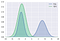Example-1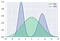Example-2

In Example-1, the mode on the right is not covered by Q(x), but P(x) > 0 on the right. Hence, Example-1 will incur high forward KL divergence and the model will be encouraged to make Q(x) assume a different form. In Example-2, Q(x) is more spread out, and covers all x where P(x) > 0. When minimizing forward KL, this is the desired result, even though Q(x) takes very high values when P(x) is small (around x = 0) as forward KL does not penalize this behavior.

Because of this, Forward KL has mean-seeking behavior, because the model’s distribution Q must cover all the modes and regions of high probability in P. Minimizing forward KL results in Q that covers all x where P(x) > 0, and hence can generate very diverse samples. But these samples are of lower quality i.e. Q can give unreasonably high probabilities to x which are very rare and implausible under P. In the following sections, we will see that the cross-entropy loss that we are so familiar with, is, in fact, equivalent to forward KL.

Reverse KL Divergence

In reverse KL, the difference between P(x) and Q(x) is weighted by Q(x). As above, we consider two cases for a training instance x:

• model thinks x not plausible and rare, Q(x) = 0. The model incurs no penalty even when x is common and plausible in the real world (P(x) > 0).
• model thinks x is more common, Q(x) > 0. In this case, the model incurs high loss when the log difference term is high. If minimizing reverse KL is used as the training objective, the difference between P(x) and Q(x) will be minimized if Q(x) > 0.

Therefore, Example-1, which was heavily penalized by forward KL is the optimal solution for reverse KL. Example-2, which is the optimal solution for forward KL is heavily penalized by reverse KL. Reverse KL encourages the model to fit a portion of P(x), as long as the fit is accurate and discourages the spreading of Q(x), as seen in Example-1.

Because of this, reverse KL Divergence has mode-seeking behavior, because any sample from the model’s distribution Q must lie within a mode of P (since it’s required that samples from Q have high probability under P). Minimizing reverse KL results can ensure that an x that has high probability under Q will definitely have a high probability under P too. Thus, samples from Q will be of higher quality. But this improvement in quality comes with a reduction in sample diversity, as Q gives zero probability to many samples which are plausible under P (P(x) > 0).

Jensen-Shannon Divergence

Both forward and reverse KL divergence have their own advantages and disadvantages. Another divergence measure that is widely used, is Jensen-Shannon Divergence (or JSD). Unlike KL Divergence, JSD is symmetric and can be understood to fall halfway between forward and reverse KL divergences.Eq. 4: Jensen-Shannon Divergence

A more general expression of JSD which allows us to arbitrarily interpolate between forward and reverse KL is given as follows:Eq. 5: Generalized Jensen-Shannon Divergence

Where 0 < π < 1. For π close to zero, it can be shown that optimizing JSπ is equivalent to optimizing forward KL divergence (KL[PQ]). Similarly, for π close to one, optimizing JSπ is equivalent to optimizing reverse KL divergence (KL[QP]). Thus, minimizing JSπ divergence for a range of π ∈ (0, 1) allows us to interpolate between the behavior of KL[PQ] and KL[QP] control the quality-diversity tradeoff.

## MLE trained models

Generative models based on RNNs/LSTMs and Transformers that are trained using Maximum Likelihood Estimation have two things in common:

• They are trained using Cross-Entropy as the loss function. The cross-entropy loss can be defined as:Eq. 6: Cross-Entropy Loss

Where p̃_{data}(x) represents the empirical data distribution (training set):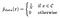Eq. 7: Empirical Data Distribution (p̃_{data}(x)), N denotes the number of training samples

and p_G represents the model’s distribution. Cross-Entropy loss minimization can be shown (below) to be equivalent to minimizing the Forward KL divergence between the empirical data distribution and the model’s distribution: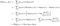Source: https://thegradient.pub/understanding-evaluation-metrics-for-language-models/

Here, H(p̃_{data}) is the entropy of p̃_{data} and D_KL(p̃_{data} ‖ p_G) is the Kullback–Leibler (KL) divergence of p_G from p̃_{data}. As the empirical entropy H(p̃_{data}) is unoptimizable, minimizing the cross-entropy loss helps us achieve the true objective of minimizing the forward KL Divergence between the empirical data distribution p̃_{data} and its approximate estimator p_G.

• They are trained using Teacher Forcing, which refers to the training procedure where the model is trained to predict the next word given previous ground truth words. However, during test time the resulting models are used to generate an entire sequence by predicting one word at a time, and by feeding the generated word back as input at the next time step.

## Sharechat

India's leading regional social media

## Sharechat

India's leading regional social media

Written by

## Kushal Chauhan

Graduate Student at Indian Institute of Information Technology and Management## Sharechat

India's leading regional social media

## More From Medium

Medium is an open platform where 170 million readers come to find insightful and dynamic thinking. Here, expert and undiscovered voices alike dive into the heart of any topic and bring new ideas to the surface. Learn more

Follow the writers, publications, and topics that matter to you, and you’ll see them on your homepage and in your inbox. Explore

If you have a story to tell, knowledge to share, or a perspective to offer — welcome home. It’s easy and free to post your thinking on any topic. Write on Medium